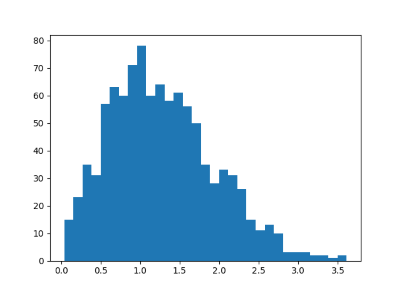# Distribution of Eigenvalues for Symmetric Gaussian Matrix

ModelingStatisticsposted by John Cook August 7, 2018

Symmetric Gaussian matrices The previous post looked at the distribution of eigenvalues for very general random matrices. In this post we will look...### Symmetric Gaussian matrices

The previous post looked at the distribution of eigenvalues for very general random matrices. In this post we will look at the eigenvalues of matrices with more structure. Fill an n by n matrix A with values drawn from a standard normal distribution and let Mbe the average of A and its transpose, i.e. M = ½(A + AT).  The eigenvalues will all be real because M is symmetric.

This is called a “Gaussian Orthogonal Ensemble” or GOE. The term is standard but a little misleading because such matrices may not be orthogonal.

### Eigenvalue distribution

The joint probability distribution for the eigenvalues of M has three terms: a constant term that we will ignore, an exponential term, and a product term. (Source)The exponential term is the same as in a multivariate normal distribution. This says the probability density drops of quickly as you go away from the origin, i.e. it’s rare for eigenvalues to be too big. The product term multiplies the distances between each pair of eigenvalues. This says it’s also rare for eigenvalues to be very close together.

(The missing constant to turn the expression above from a proportionality to an equation is whatever it has to be for the right side to integrate to 1. When trying to qualitatively understand a probability density, it usually helps to ignore proportionality constants. They are determined by the rest of the density expression, and they’re often complicated.)

If eigenvalues are neither tightly clumped together, nor too far apart, we’d expect that the distance between them has a distribution with a hump away from zero, and a tail that decays quickly. We will demonstrate this with a simulation, then give an exact distribution.

### Python simulation

The following Python code simulates 2 by 2 Gaussian matrices.

    import matplotlib.pyplot as plt
import numpy as np

n = 2
reps = 1000

diffs = np.zeros(reps)
for r in range(reps):
A = np.random.normal(scale=n**-0.5, size=(n,n))
M = 0.5*(A + A.T)
w = np.linalg.eigvalsh(M)
diffs[r] = abs(w - w)

plt.hist(diffs, bins=int(reps**0.5))
plt.show()


This produced the following histogram:The exact probability distribution is p(s) = s exp(-s²/4)/2. This result is known as “Wigner’s surmise.”

Original Source## John Cook

Companies come to me for help with probability, machine learning, mathematical modeling, … anything that falls under applied math, statistics, and computing. Clients have included large companies such as Amazon, Google, Microsoft, and Amgen, as well as a number of law firms, start-ups, and smaller businesses. I help companies take advantage of their data by combining it with expert opinion, uncovering latent insights, creating mathematical models, overcoming computational difficulties, and interpreting the results.

1